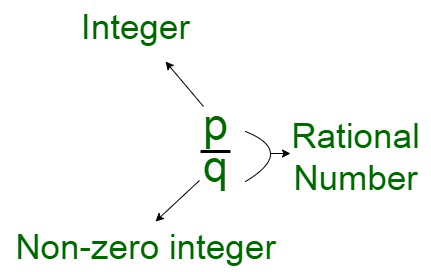Open in App
Not now

# How many rational numbers are there between 0 and 1?

• Difficulty Level : Easy
• Last Updated : 30 Nov, 2021

Number System is a systematic way of representation of numbers of the number line. These numbers are depicted using a set of symbols and rules. The numbers lie in the interval belonging from 0-9. These numbers are also known as digits and can be manipulated using various mathematical operations. All the computations like counting, inputting, and manipulating can be performed using calculations of the number line.

Rational Numbers

A rational number is a number that is denoted in the form of a fraction, p/q where p and q are integers and also, q is not equal to 0. The entire set of rational numbers in the number system is denoted by the letter Q. In other words, In case, a number can be expressed as a fraction where both the numerator and the denominator are integral values, the number is called a rational number. These rational numbers can also be simplified further to obtain pure whole numbers or decimal values.Examples of Rational Numbers

Some of the examples of rational numbers can be:

• 1/2
• -3/4
• 0.3 or 3/10
• -0.7 or -7/10
• 0.141414… or 14/99

### How many rational numbers are there between 0 and 1?

Solution:

There are infinite sets of rational numbers contained between 0 and 1. To prove this, let us consist of an interval,

[0 , 1]

Now dividing it into two parts, we obtain, [0,1/2] and [1/2, 1]

Taking A as [0,1/2], we can further divide it into [0, 1/4] and [1/4, 1/2]

Taking A’ as [0,1/4], we can further divide it into [0, 1/8] and [1/8, 1/4]

Similarly, we can infinitely divide these intervals exponentially into further smaller intervals.

There is no upper bound to the number of intervals that can be created. Each of the obtained intervals at least adds one number to the count of rational numbers between 0 and 1.

Hence, there are infinite rational numbers between 0 and 1.

Extending this argument further, we get,

Note: Between any two real numbers a and b, there are an infinite number of real numbers.

### Similar Questions

Question 1. Find out a rational number between 2/3 and 3/4?

Solution:

To find a rational number between 2/3 and 3/4

Find the average of these two rational numbers

Average = (2/3 + 3/4)/2

LCM of 3 and 4 = 12

Average = ((4×2)+(3×3)/12)/2

Average = (8+9/12)/2

Average = 17/12/2

Average = 17/12 × 1/2

Average = 17/24

Therefore,

The rational number between 2/3 and 3/4 is 17/24.

Question 2. Find out the rational number from these fractions;

1/7, 99/0, 7/6, 0, 2/7, 5/8, 5.

Solution:

Here we have to find out the rational numbers

1/7, 99/0, 7/6, 0, 2/7, 5/8, 5.

As we know that

Rational numbers are in the form of a/b, where b ≠ 0

Therefore,

1/7 is a rational number

7/6 is a rational number

2/7 is a rational number

5/8 is a rational number

Question 3. Find five rational numbers between 1/2 and 2/3?

Solution:

First make the denominators same

Taking LCM of 2 and 3

LCM of 2 and 3 = 6

Now the rational numbers will be

1×3/2×3 and 2×2/3×2

3/6 and 4/6

Now multiply both numerator and denominator by 10 of both the rational numbers

3×10/6×10 = 30/60

4×10/6×10 = 40/60

Now,

The rational numbers between 1/2 and 2/3 will be

31/60, 32/60, 33/60, 34/60, 35/60

Question 4. Write any ten rational numbers between -4/5 and 6/7?

Solution:

First find the LCM of 5 and 7

LCM of 5 and 7 = 35

Now,

-4×7/5×7 = -28/35

6×5/7 ×5 = 30/35

Thus,

The rational numbers between -4/5 and 6/7 are -27/35, -26/35, -25/35, -24/35, -23/35, -22/35, -21/35, -20/35, -19/35, -18/35,….., 29/35.

My Personal Notes arrow_drop_up